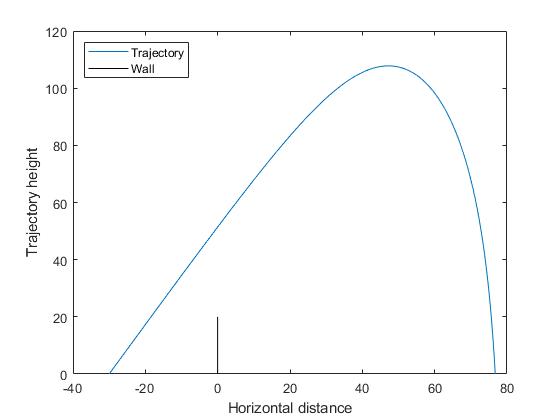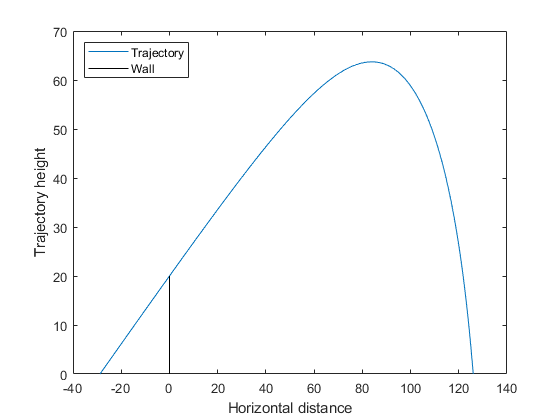Documentation

Optimize an ODE in Parallel

This example shows how to optimize parameters of an ODE.

It also shows how to avoid computing the objective and nonlinear constraint function twice when the ODE solution returns both. The example compares patternsearch and ga in terms of time to run the solver and the quality of the solutions.

You need a Parallel Computing Toolbox™ license to use parallel computing.

Step 1. Define the problem.

The problem is to change the position and angle of a cannon to fire a projectile as far as possible beyond a wall. The cannon has a muzzle velocity of 300 m/s. The wall is 20 m high. If the cannon is too close to the wall, it has to fire at too steep an angle, and the projectile does not travel far enough. If the cannon is too far from the wall, the projectile does not travel far enough either.

Air resistance slows the projectile. The resisting force is proportional to the square of the velocity, with proportionality constant 0.02. Gravity acts on the projectile, accelerating it downward with constant 9.81 m/s2. Therefore, the equations of motion for the trajectory x(t) are

$\frac{{d}^{2}x\left(t\right)}{d{t}^{2}}=-0.02‖v\left(t\right)‖v\left(t\right)-\left(0,9.81\right),$

where $v\left(t\right)\text{​}=dx\left(t\right)/dt.$

The initial position x0 and initial velocity xp0 are 2-D vectors. However, the initial height x0(2) is 0, so the initial position depends only on the scalar x0(1). And the initial velocity xp0 has magnitude 300 (the muzzle velocity), so depends only on the initial angle, a scalar. For an initial angle th, xp0 = 300*(cos(th),sin(th)). Therefore, the optimization problem depends only on two scalars, so it is a 2-D problem. Use the horizontal distance and the angle as the decision variables.

Step 2. Formulate the ODE model.

ODE solvers require you to formulate your model as a first-order system. Augment the trajectory vector (x1(t),x2(t)) with its time derivative (x'1(t),x'2(t)) to form a 4-D trajectory vector. In terms of this augmented vector, the differential equation becomes

$\frac{d}{dt}x\left(t\right)=\left[\begin{array}{c}{x}_{3}\left(t\right)\\ {x}_{4}\left(t\right)\\ -.02‖\left({x}_{3}\left(t\right),{x}_{4}\left(t\right)\right)‖{x}_{3}\left(t\right)\\ -.02‖\left({x}_{3}\left(t\right),{x}_{4}\left(t\right)\right)‖{x}_{4}\left(t\right)-9.81\end{array}\right].$

Write the differential equation as a function file, and save it on your MATLAB® path.

function f = cannonfodder(t,x)

f = [x(3);x(4);x(3);x(4)]; % initial, gets f(1) and f(2) correct
nrm = norm(x(3:4)) * .02; % norm of the velocity times constant
f(3) = -x(3)*nrm; % horizontal acceleration
f(4) = -x(4)*nrm - 9.81; % vertical acceleration

Visualize the solution of the ODE starting 30 m from the wall at an angle of pi/3.Step 3. Solve using patternsearch.

The problem is to find initial position x0(1) and initial angle x0(2) to maximize the distance from the wall the projectile lands. Because this is a maximization problem, minimize the negative of the distance (see Maximizing vs. Minimizing).

To use patternsearch to solve this problem, you must provide the objective, constraint, initial guess, and options.

These two files are the objective and constraint functions. Copy them to a folder on your MATLAB path.

function f = cannonobjective(x)
x0 = [x(1);0;300*cos(x(2));300*sin(x(2))];

sol = ode45(@cannonfodder,[0,15],x0);

% Find the time t when y_2(t) = 0
zerofnd = fzero(@(r)deval(sol,r,2),[sol.x(2),sol.x(end)]);
% Then find the x-position at that time
f = deval(sol,zerofnd,1);

f = -f; % take negative of distance for maximization

function [c,ceq] = cannonconstraint(x)

ceq = [];
x0 = [x(1);0;300*cos(x(2));300*sin(x(2))];

sol = ode45(@cannonfodder,[0,15],x0);

if sol.y(1,end) <= 0 % projectile never reaches wall
c = 20 - sol.y(2,end);
else
% Find when the projectile crosses x = 0
zerofnd = fzero(@(r)deval(sol,r,1),[sol.x(2),sol.x(end)]);
% Then find the height there, and subtract from 20
c = 20 - deval(sol,zerofnd,2);
end

Notice that the objective and constraint functions set their input variable x0 to a 4-D initial point for the ODE solver. The ODE solver does not stop if the projectile hits the wall. Instead, the constraint function simply becomes positive, indicating an infeasible initial value.

The initial position x0(1) cannot be above 0, and it is futile to have it be below –200. (It should be near –20 because, with no air resistance, the longest trajectory would start at –20 at an angle pi/4.) Similarly, the initial angle x0(2) cannot be below 0, and cannot be above pi/2. Set bounds slightly away from these initial values:

lb = [-200;0.05];
ub = [-1;pi/2-.05];
x0 = [-30,pi/3]; % initial guess

Set the UseCompletePoll option to true. This gives a higher-quality solution, and enables direct comparison with parallel processing, because computing in parallel requires this setting.

opts = optimoptions('patternsearch','UseCompletePoll',true);

Call patternsearch to solve the problem.

tic % time the solution
[xsolution,distance,eflag,outpt] = patternsearch(@cannonobjective,x0,...
[],[],[],[],lb,ub,@cannonconstraint,opts)
toc
Optimization terminated: mesh size less than options.MeshTolerance
and constraint violation is less than options.ConstraintTolerance.

xsolution =
-28.8123    0.6095

distance =
-125.9880

eflag =
1

outpt =
function: @cannonobjective
problemtype: 'nonlinearconstr'
pollmethod: 'gpspositivebasis2n'
maxconstraint: 0
searchmethod: []
iterations: 5
funccount: 269
meshsize: 8.9125e-07
rngstate: [1x1 struct]
message: 'Optimization terminated: mesh size less than options.MeshTolerance…'

Elapsed time is 3.174088 seconds.

Starting the projectile about 29 m from the wall at an angle 0.6095 radian results in the farthest distance, about 126 m. The reported distance is negative because the objective function is the negative of the distance to the wall.

Visualize the solution.

x0 = [xsolution(1);0;300*cos(xsolution(2));300*sin(xsolution(2))];

sol = ode45(@cannonfodder,[0,15],x0);
% Find the time when the projectile lands
zerofnd = fzero(@(r)deval(sol,r,2),[sol.x(2),sol.x(end)]);
t = linspace(0,zerofnd); % equal times for plot
xs = deval(sol,t,1); % interpolated x values
ys = deval(sol,t,2); % interpolated y values
plot(xs,ys)
hold on
plot([0,0],[0,20],'k') % Draw the wall
xlabel('Horizontal distance')
ylabel('Trajectory height')
legend('Trajectory','Wall','Location','NW')
ylim([0 70])
hold offStep 4. Avoid calling the expensive subroutine twice.

Both the objective and nonlinear constraint function call the ODE solver to calculate their values. Use the technique in Objective and Nonlinear Constraints in the Same Function (Optimization Toolbox) to avoid calling the solver twice. The runcannon file implements this technique. Copy this file to a folder on your MATLAB path.

function [x,f,eflag,outpt] = runcannon(x0,opts)

if nargin == 1 % No options supplied
opts = [];
end

xLast = []; % Last place ode solver was called
sol = []; % ODE solution structure

fun = @objfun; % the objective function, nested below
cfun = @constr; % the constraint function, nested below

lb = [-200;0.05];
ub = [-1;pi/2-.05];

% Call patternsearch
[x,f,eflag,outpt] = patternsearch(fun,x0,[],[],[],[],lb,ub,cfun,opts);

function y = objfun(x)
if ~isequal(x,xLast) % Check if computation is necessary
x0 = [x(1);0;300*cos(x(2));300*sin(x(2))];
sol = ode45(@cannonfodder,[0,15],x0);
xLast = x;
end
% Now compute objective function
% First find when the projectile hits the ground
zerofnd = fzero(@(r)deval(sol,r,2),[sol.x(2),sol.x(end)]);
% Then compute the x-position at that time
y = deval(sol,zerofnd,1);
y = -y; % take negative of distance
end

function [c,ceq] = constr(x)
ceq = [];
if ~isequal(x,xLast) % Check if computation is necessary
x0 = [x(1);0;300*cos(x(2));300*sin(x(2))];
sol = ode45(@cannonfodder,[0,15],x0);
xLast = x;
end
% Now compute constraint functions
% First find when the projectile crosses x = 0
zerofnd = fzero(@(r)deval(sol,r,1),[sol.x(1),sol.x(end)]);
% Then find the height there, and subtract from 20
c = 20 - deval(sol,zerofnd,2);
end

end

Reinitialize the problem and time the call to runcannon.

x0 = [-30;pi/3];
tic
[xsolution,distance,eflag,outpt] = runcannon(x0,opts);
toc
Elapsed time is 2.610590 seconds.

The solver ran faster than before. If you examine the solution, you see that the output is identical.

Step 5. Compute in parallel.

Try to save more time by computing in parallel. Begin by opening a parallel pool of workers.

parpool
Starting parpool using the 'local' profile ... connected to 4 workers.

ans =

Pool with properties:

Connected: true
NumWorkers: 4
Cluster: local
AttachedFiles: {}
IdleTimeout: 30 minute(s) (30 minutes remaining)
SpmdEnabled: true

Set the options to use parallel computing, and rerun the solver.

opts = optimoptions('patternsearch',opts,'UseParallel',true);
x0 = [-30;pi/3];
tic
[xsolution,distance,eflag,outpt] = runcannon(x0,opts);
toc
Elapsed time is 3.917971 seconds.

In this case, parallel computing was slower. If you examine the solution, you see that the output is identical.

Step 6. Compare with the genetic algorithm.

You can also try to solve the problem using the genetic algorithm. However, the genetic algorithm is usually slower and less reliable.

The runcannonga file calls the genetic algorithm and avoids double evaluation of the ODE solver. It resembles runcannon, but calls ga instead of patternsearch, and also checks whether the trajectory reaches the wall. Copy this file to a folder on your MATLAB path.

function [x,f,eflag,outpt] = runcannonga(opts)

if nargin == 1 % No options supplied
opts = [];
end

xLast = []; % Last place ode solver was called
sol = []; % ODE solution structure

fun = @objfun; % the objective function, nested below
cfun = @constr; % the constraint function, nested below

lb = [-200;0.05];
ub = [-1;pi/2-.05];

% Call ga
[x,f,eflag,outpt] = ga(fun,2,[],[],[],[],lb,ub,cfun,opts);

function y = objfun(x)
if ~isequal(x,xLast) % Check if computation is necessary
x0 = [x(1);0;300*cos(x(2));300*sin(x(2))];
sol = ode45(@cannonfodder,[0,15],x0);
xLast = x;
end
% Now compute objective function
% First find when the projectile hits the ground
zerofnd = fzero(@(r)deval(sol,r,2),[sol.x(2),sol.x(end)]);
% Then compute the x-position at that time
y = deval(sol,zerofnd,1);
y = -y; % take negative of distance
end

function [c,ceq] = constr(x)
ceq = [];
if ~isequal(x,xLast) % Check if computation is necessary
x0 = [x(1);0;300*cos(x(2));300*sin(x(2))];
sol = ode45(@cannonfodder,[0,15],x0);
xLast = x;
end
% Now compute constraint functions
if sol.y(1,end) <= 0 % projectile never reaches wall
c = 20 - sol.y(2,end);
else
% Find when the projectile crosses x = 0
zerofnd = fzero(@(r)deval(sol,r,1),[sol.x(2),sol.x(end)]);
% Then find the height there, and subtract from 20
c = 20 - deval(sol,zerofnd,2);
end
end

end

Call runcannonga in parallel.

opts = optimoptions('ga','UseParallel',true);
rng default % for reproducibility
tic
[xsolution,distance,eflag,outpt] = runcannonga(opts)
toc
Optimization terminated: average change in the fitness value less than
options.FunctionTolerance and constraint violation is less than options.ConstraintTolerance.

xsolution =
-17.9172    0.8417

distance =
-116.6263

eflag =
1

outpt =
problemtype: 'nonlinearconstr'
rngstate: [1x1 struct]
generations: 5
funccount: 20212
message: [1x140 char]
maxconstraint: 0

Elapsed time is 119.630284 seconds.

The ga solution is not as good as the patternsearch solution: 117 m versus 126 m. ga took much more time: about 120 s versus under 5 s.